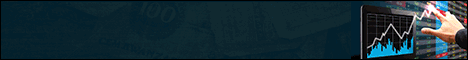Hello Guest, if you are reading this it means you have not registered yet. Please take a second, Click here to register, and in a few simple steps you will be able to enjoy all the many features of our fine community. Note that lewd or meaningless nicknames are prohibited (no numbers or letters at random) and please introduce yourself in the section for you to meet our community.

# Thread: How Indicators are calculated?

1. ## How Indicators are calculated?

Dear members
Hi,

Here we are going to introduce and talk about how some the most useful indicators are calculated and may be discuss about how they are being used in trading.

Be healthy & wealthy
News DeskReply With Quote

2. ## EMA - Exponential Moving Average

EMA - Exponential Moving Average

Exponetial Moving Average (EMA for short) is one of the most used indicators in technical analysis today. But how do you calculate it for yourself, using a paper and a pen or – preferred – a spreadsheet program of your choice. Let’s find out in this explanation of EMA calculation.

Calculating Exponential Moving Average (EMA) is of course done automatically by most trading and technical analysis software out there today.

Here is how to calculate it manually which also adds to the understanding on how it works.

In this example we shall calculate EMA for a the price of a stock. We want a 22 day EMA which is a common enough time frame for a long EMA.

The formula for calculating EMA is as follows:

EMA = Price(t) * k + EMA(y) * (1 – k)
t = today, y = yesterday, N = number of days in EMA, k = 2/(N+1)

Use the following steps to calculate a 22 day EMA:

1) Start by calculating k for the given time frame. 2 / (22 + 1) = 0,0869

2) Add the closing prices for the first 22 days together and divide them by 22.

3) You’re now ready to start getting the first EMA day by taking the following day’s (day 23) closing price multiplied by k, then multiply the previous day’s moving average by (1-k) and add the two.

4) Do step 3 over and over for each day that follows to get the full range of EMA.

This can of course be put into Excel or some other spreadsheet software to make the process of calculating EMA semi-automatic.

GoodluckReply With Quote

3.Originally Posted by PCMNewsdeskEMA - Exponential Moving Average

Exponetial Moving Average (EMA for short) is one of the most used indicators in technical analysis today. But how do you calculate it for yourself, using a paper and a pen or – preferred – a spreadsheet program of your choice. Let’s find out in this explanation of EMA calculation.

Calculating Exponential Moving Average (EMA) is of course done automatically by most trading and technical analysis software out there today.

Here is how to calculate it manually which also adds to the understanding on how it works.

In this example we shall calculate EMA for a the price of a stock. We want a 22 day EMA which is a common enough time frame for a long EMA.

The formula for calculating EMA is as follows:

Use the following steps to calculate a 22 day EMA:

1) Start by calculating k for the given time frame. 2 / (22 + 1) = 0,0869

2) Add the closing prices for the first 22 days together and divide them by 22.

3) You’re now ready to start getting the first EMA day by taking the following day’s (day 23) closing price multiplied by k, then multiply the previous day’s moving average by (1-k) and add the two.

4) Do step 3 over and over for each day that follows to get the full range of EMA.

This can of course be put into Excel or some other spreadsheet software to make the process of calculating EMA semi-automatic.

Goodluck

What about other indicators. Though everything can be found in the wikipedia I would like to get easier and clearer explanation here. Now I trade with MACD and bollinger bands with my broker. Can you explain their origination and ground principles they work on?Reply With Quote

4. Indicators. Indicators are composed of multiple data elements, and typically consist of a numerator and denominator. Calculated totals do not have a denominator. Indicators are used to calculate coverage rates, incidence and other values are a result of data element values that have been entered into the system.Reply With Quote

5. Hi,
First of all thanks for sharing this valuable knowledge.
I am new on forex trading. I want to know more about forex.

""2) Add the closing prices for the first 22 days together and divide them by 22.

3) You’re now ready to start getting the first EMA day by taking the following day’s (day 23) closing price multiplied by k, then multiply the previous day’s moving average by (1-k) and add the two""

Actually i understand step 1. But in step two you said devided them by 22, this term i don't understand. And what is the full definition of (k).

Thank you..Reply With QuoteReply With Quote

7. How i calculate my profit perfectly. Does any body know?Reply With Quote

8.####Posting Permissions

• You may not post new threads
• You may not post replies
• You may not post attachments
• You may not edit your posts
•Credits System provided by vBCredits II Deluxe v2.1.1 (Pro) - vBulletin Mods & Addons Copyright © 2020 DragonByte Technologies Ltd.